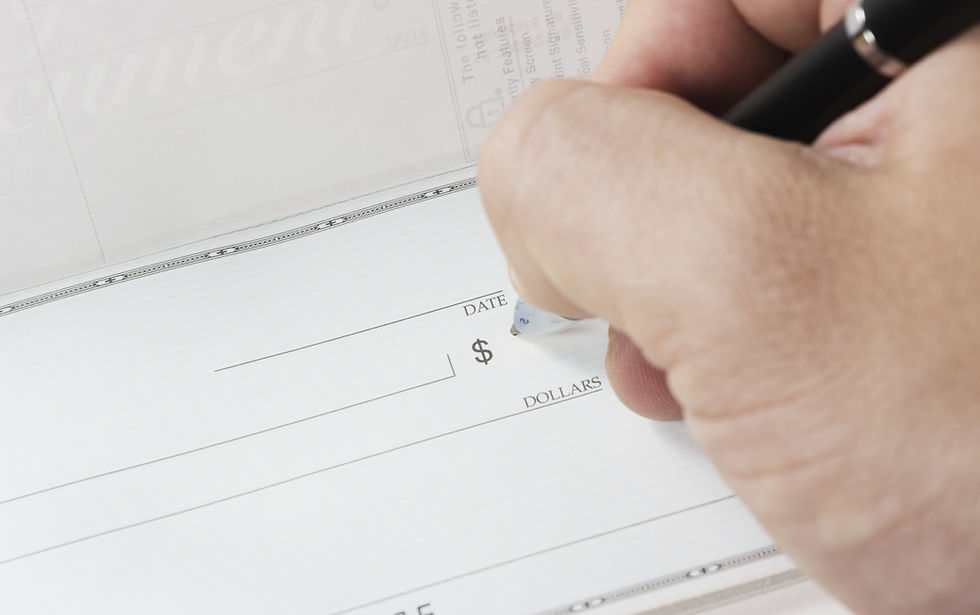top of page
Search

# Gordon's Dividend Growth Model - ACCA FM Technical Article

Updated: Aug 23, 2020

Gordon's Dividend Growth Model, or Dividend Growth Model (DGM), is a popular topic in ACCA Financial Management (ACCA FM or ACCA F9) exam.

In the study text, you will find DGM in two subject areas which are -

1. Business Finance: Apply DGM formula to estimate cost of equity;

2. Business Valuation: Apply DGM formula to value a company's equity.

In fact, the same formula will different interpretation can help to derive answers for different purposes.

I would like to share with you on applying Dividend Growth Model in business valuation by explaining the concepts and demonstrating how to apply the formula in getting company's equity value with a sample ACCA question.### Equity Value

To valuate a company's equity value, there are several approaches can be adopted. One of the commonly used model is dividend growth model.

FormulaWhere,

re = cost of equity

D0 = current dividend

P0 = ex –dividend share price

g = the expected annual constant rate of dividend growth

Q: Which area of growth model relevant?

A: Dividend growth model is relevant under business finance.

Q: What is growth model?

A: It is 1 of methods to find rate of return that investors require to persuade them to invest in company’s ordinary shares.

Q: What is the limitation of growth model?

A: 1 of key assumptions is growth rate cannot exceed cost of equity. This presumption of a steady and perpetual growth rate less than the cost of capital may not be reasonable.### Illustration

Learning concepts without applying them are not the best way to study.

I pick one question from ACCA Financial Management Specimen paper 2016 to show how to get the equity valuation number.The question asks about the market price of SKV Co shares, so the following DGM formula applies -

P0 = D0 (1 + g) / (re - g)

How do I work on this question? I will do it in the following steps -

1. Find the growth rate (g) first;

2. Apply DGM formula by putting growth rate, dividend and cost of equity;

3. Share price is found.

Step 1 - Finding growth rate

Dividend is grown from \$0.311/share in 20X1 to \$0.360/share in 20X4, therefore, geometric average growth rate is -

(0.360 / 0.311)^(1/3) - 1

Note: From 20X1 to 20X4 is 3 years

Dividend growth rate is 4.998% or 5%

Step 2 - Put all parameters in DGM formula

Given current dividend (20X4) is \$0.360/share, cost of equity is 12% and growth rate calculated is 5%, putting all these into DGM formula -

P0 = 0.360 x (1 + 5%) / (12% - 5%)

Step 3 - Finding share price

With the formula above, it is found the share price is \$5.40.

The correct answer is Option C.### Conclusion

One of challenging part in adopting Dividend Growth Model in valuing a company's equity is finding growth rate.

We show you how to find the growth rate in the example while you should know how to do it in exam.

In addition to that, please be reminded the share price in formula should be ex-dividend share price.

If you would like to try more questions, just visit our Practice Question and Mock Exam sections.